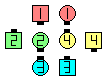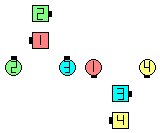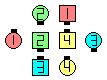Definitions of Square Dance Calls and Concepts

Index -->  Plus  |  A1  |  A2  |  C1  |  C2  |  C3A  |  C3B  |  C4  |  NOL  |
Definitions (Text Only) -->  Plus  |  A1  |  A2  |  C1  |  C2  |  C3A  |  C3B  |  C4  |  NOL  |
 Find call:

 \$B8@8l(B1/4 Tag \$B\$+\$i(B.

Flip Your Lid \$B\$K\$O(B 4 \$B\$D\$N0[\$J\$C\$?F0:n\$,\$"\$j\$^\$9(B:

 Center Wave \$B\$N(B Ends: \$BE,@Z\$J(B outside \$B\$N?M\$H(B Scoot Back \$B\$r\$7\$^\$9(B; Very Center: Centers Run \$B\$N<+J,\$N%Q!<%H\$r\$7(B & Circle 1/4 \$B\$r\$7\$^\$9(B (Scoot Back \$B\$r\$9\$k\$N\$G\$O\$J\$\$\$3\$H\$KCm0U(B); Outside Belle (\$B1&\$N(B 1/4 Tag \$B\$N\$H\$-(B) \$B\$^\$?\$O(B Outside Beau (\$B:8\$N(B 1/4 Tag \$B\$N\$H\$-(B): Scoot Back \$B\$N<+J,\$N%Q!<%H\$r\$7(B & Circle 1/4 \$B\$r\$7\$^\$9(B (\$BCf\$r8~\$\$\$F=*\$o\$j\$^\$9(B). \$BB>\$N(B Outside \$B\$N?M(B: Press Ahead, Trade (opposite \$B\$N?M\$H(B), & Spread \$B\$r\$7\$^\$9(B.

1/4 Tag \$B\$G=*\$o\$j\$^\$9(B.Flip Your Lid\$B\$NA0(B part-way \$B8e(B

\$B%@%s%9\$N%R%s%H(B:

• Center Wave \$B\$N(B Ends \$B\$ONY\$N(B center \$B\$N?M\$r(B \$B>/\$7\$@\$1(B\$B!!0z\$/(B (Recycle \$B\$d(B Flip Back \$B\$N\$H\$-\$N\$h\$&\$K(B) \$B\$H(B, \$B@5\$7\$\$J}\$XF0\$-;O\$a\$k\$3\$H\$NChoreography for Flip Your LidComments? Questions? Suggestions?